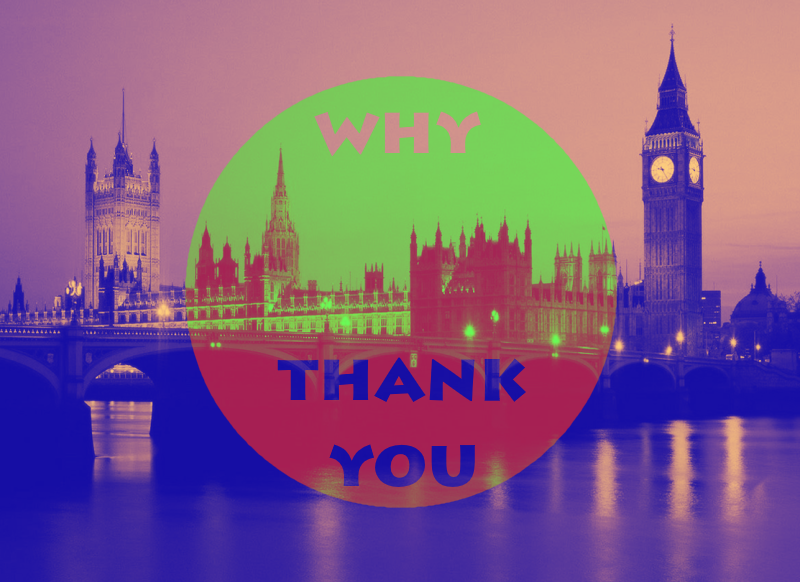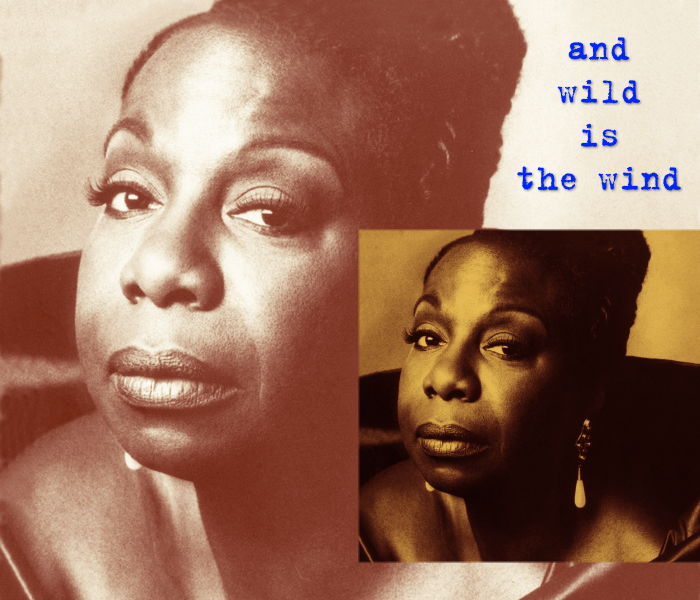## Recommended Posts

Back with my second plugin. This time I made a plugin that can be used to easily and quickly create a gradient mapping effect using 2 colors. I know pyrochild already made a gradient mapping tool, but this is just a simplified version using only 2 colors for dark and light tones. I added some options for post processing as well, like contrast and blending mode.

Preview:Code: (I’m still a beginner in C#, please don’t hurt me)

```// Name: Duotone Gradient Map
// Author: Pascal
// Version: 1.0
// Desc: Easy and quick way of gradient mapping
// Keywords:
// URL:
// Help:
#region UICode
ColorWheelControl Amount1 = ColorBgra.FromBgr(0, 0, 0); // Dark
ColorWheelControl Amount2 = ColorBgra.FromBgr(255, 255, 255); // Light
IntSliderControl Amount3 = 100; // [0,100] Amount
IntSliderControl Amount4 = 0; // [0,1000] Contrast
ListBoxControl Amount5 = 0; // Blending mode|Normal|Multiply|Darken|Lighten|Additive
#endregion

void Render(Surface dst, Surface src, Rectangle rect)
{
ColorBgra Primary = Amount1;
ColorBgra Secondary = Amount2;
ColorBgra Cp; //current pixel
ColorBgra Np; //new pixel
for (int y = rect.Top; y < rect.Bottom; y++)
{
if (IsCancelRequested) return;
for (int x = rect.Left; x < rect.Right; x++)
{
Cp = src[x,y];

//control parameters
double A = ((double)Amount3)/100; //amount
double C = (double)Amount4; //contrast
int M = Amount5; //blending mode

//get rgb
double R = Cp.R;
double G = Cp.G;
double B = Cp.B;

//value
double P = ((R+G+B)/3)/255; //luminosity

//get colors
double Rp = Primary.R;
double Rs = Secondary.R;
double Gp = Primary.G;
double Gs = Secondary.G;
double Bp = Primary.B;
double Bs = Secondary.B;

//calculate new color
R = Norm((P*Rs + (1-P)*Rp)*A + (1-A)*R, 255);
G = Norm((P*Gs + (1-P)*Gp)*A + (1-A)*G, 255);
B = Norm((P*Bs + (1-P)*Bp)*A + (1-A)*B, 255);

//apply new color and contrast
Np = ColorBgra.FromBgra(
(byte)Norm(Con(B, C), 255),
(byte)Norm(Con(G, C), 255),
(byte)Norm(Con(R, C), 255),
Cp.A);

//BLENDING MODES

//multiply
if(M == 1){
Cp.R = (byte)Norm((Cp.R * Np.R/255)*A + Cp.R*(1-A), 255);
Cp.G = (byte)Norm((Cp.G * Np.G/255)*A + Cp.G*(1-A), 255);
Cp.B = (byte)Norm((Cp.B * Np.B/255)*A + Cp.B*(1-A), 255);
}
//darken
else if(M == 2){
if(Np.R < Cp.R){
Cp.R = Np.R;
}
if(Np.G < Cp.G){
Cp.G = Np.G;
}
if(Np.B < Cp.B){
Cp.B = Np.B;
}
}
//lighten
else if(M == 3){
if(Np.R > Cp.R){
Cp.R = Np.R;
}
if(Np.G > Cp.G){
Cp.G = Np.G;
}
if(Np.B > Cp.B){
Cp.B = Np.B;
}
}
else if(M == 4){
Cp.R = (byte)Norm(Cp.R + Np.R*A, 255);
Cp.G = (byte)Norm(Cp.G + Np.G*A, 255);
Cp.B = (byte)Norm(Cp.B + Np.B*A, 255);

}
//normal
else{
Cp = Np;
}

dst[x,y] = Cp;
}
}
}

//normalize
double Norm(double x, int i){
if(x > i){
x = i;
}
if(x < 0){
x = 0;
}
return x;
}

//contrast
double Con(double x, double c){
x /= 255;
double y;
if(x <= 0.5){
y = 0.5 * Math.Pow(2*x, (c/500)+1);
}
else{
y = 1 - 0.5 * Math.Pow(2 - 2*x, (c/500)+1);
}
return y*255;
}```

•2
•1
•1
##### Share on other sites

```//BLENDING MODES

//multiply
if(M == 1){```

You could clean up the code using Switch /Case for these tests.

```      switch (M)
{
case 1:
//Console.WriteLine("Case 1");
break;
case 2:
//Console.WriteLine("Case 2");
break;

...

default:
//Console.WriteLine("Default case");
break;
}```

It's not a big thing - but BoltBait & toe_head2001 have got some awesome coloring effects for sliders baked right into Codelab. They look really coolebook: Mastering Paint.NET | resources: Plugin Index | Stereogram Tut | proud supporter of Codelab
plugins: EER's Plugin Pack | Planetoid | StickMan | WhichSymbol+ | Dr Scott's Markup Renderer | CSV Filetype | dwarf horde plugins: Plugin Browser | ShapeMaker

##### Share on other sites

1 hour ago, Ego Eram Reputo said:

You could clean up the code using Switch /Case for these tests.

Using an enum is even cleaner.```#region UICode
ListBoxControl<BlendingMode> blendingMode = 0; // Blending mode|Normal|Multiply|Darken|Lighten|Additive
#endregion

enum BlendingMode
{
Normal,
Multiply,
Darken,
Lighten,
}

void Render(Surface dst, Surface src, Rectangle rect)
{
switch (blendingMode)
{
case BlendingMode.Multiply:
// code here
break;

case BlendingMode.Darken:
// code here
break;

case BlendingMode.Lighten:
// code here
break;

// code here
break;

case BlendingMode.Normal:
default:
// code here
break;
}
}```

•1
•2My Gallery  |  My Plugin Pack

##### Share on other sites

I wanted to make a plugin very similar to this at one time. Awesome plugin!##### Share on other sites

• 4 months later...

Works like charm @pascal. Endless possibilities to recolor photo - and very good settings.my gallery is  here

##### Share on other sites

@pascal - Nice. Whilst the "suggestions" are constructive, you've built some code that can be used. There's always a critic. Your code is entirely readable. Stick with what you feel comfortable with.

## Join the conversation

You can post now and register later. If you have an account, sign in now to post with your account.×   Pasted as rich text.   Paste as plain text instead

Only 75 emoji are allowed.

×   Your previous content has been restored.   Clear editor

×   You cannot paste images directly. Upload or insert images from URL.

×
×